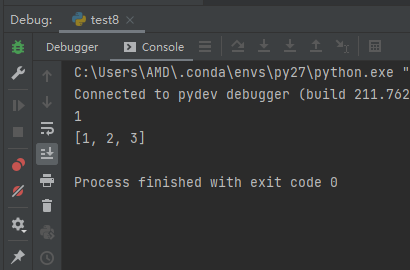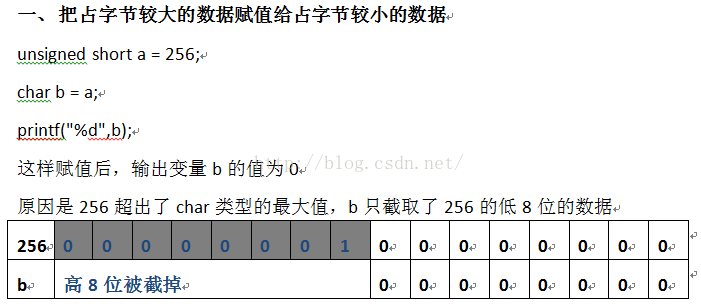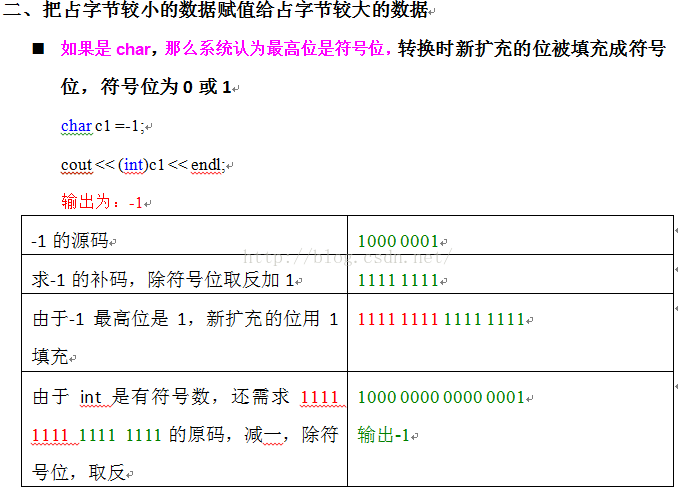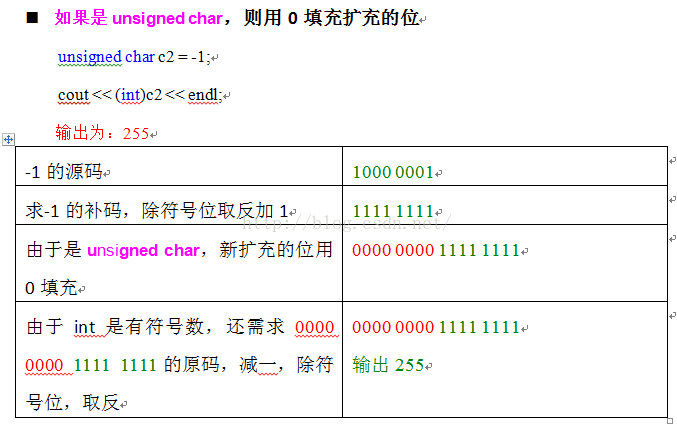• 数组压缩，将普通数组稀疏 Statistics类（稀疏数组） public class Statistics { int line; int col=3; int[][] array; public Statistics(OriArray ori){ //直接在构造器中根据原数组开辟稀疏后数组需要的空间...
数组压缩，将普通数组稀疏
Statistics类（稀疏数组）
public class Statistics {
int line;
int col=3;
int[][] array;
public Statistics(OriArray ori){  //直接在构造器中根据原数组开辟稀疏后数组需要的空间然后稀疏数组
int li=1;
int i,j,num=0;
for(i=0;i<ori.line;i++)
for(j=0;j<ori.col;j++)
if(ori.array[i][j]!=0)
num++;
this.array = new int[num+1][col];
this.line=num+1;
this.array=ori.line;
this.array=ori.col;
for(i=0;i<ori.line;i++)
for(j=0;j<ori.col;j++)
if(ori.array[i][j]!=0){
this.array[li]=i;
this.array[li]=j;
this.array[li]=ori.array[i][j];
li++;
}
}
public void prin(){		//输出数组
int i,j;
System.out.println("----------------------------");
System.out.println("压缩数组为：");
System.out.println(this.array+" "+this.array);
for(i=1;i<this.line;i++) {
for (j = 0; j < this.col; j++)
System.out.print(this.array[i][j]+" ");
System.out.println();
}
}
}

OriArriay类（创建原数组）
import java.util.Scanner;

public class OriArray {
int line;
int col;
int[][] array;
Scanner scan = new Scanner(System.in);
public void create(){			//根据需求创建原数组
int i,j;
System.out.print("请输入您需要的行数：");
this.line = scan.nextInt();
System.out.print("请输入您需要的列数：");
this.col = scan.nextInt();
array = new int[line][col];
System.out.print("现在请您输入一个"+line+"行"+col+"列的数组：");
for(i=0;i<line;i++)
for(j=0;j<col;j++)
array[i][j] = scan.nextInt();
}
public void prin(){		//输出原数组
int i,j;
System.out.println("----------------------------");
System.out.println("原数组为：");
for(i=0;i<line;i++) {
for (j = 0; j < col; j++)
System.out.print(array[i][j] + "  ");
System.out.println();
}
}
}

展开全文• 首先认识一下什么是一维数组什么是二维数组： ...二维数组压缩为一维数组： a = [[1,2,3],[4,5,6],[7,8,9]] c = [] for i in range(len(a)): for j in range(len(a[i])): b = a[i][j] c.append(b) print
首先认识一下什么是一维数组什么是二维数组：
a1 = [1,2,3,4,5,6]
a2 = [[1,2,3],[4,5,6],[7,8,9]]

print a1
print a2

输出结果：二维数组压缩为一维数组：
a = [[1,2,3],[4,5,6],[7,8,9]]

c = []
for i in range(len(a)):
for j in range(len(a[i])):
b = a[i][j]
c.append(b)
print c

二维数组a压缩为一维数组c
数组创建方法：
通过np创建（np为python库numpy）
import numpy as np

#创建并且赋值一维数组
array = np.zeros(3)
array = 1
array = 2
array = 3
print array

#创建并且赋值二维数组
narray = np.zeros((3,2))
narray = 1
narray = 3
print narray

通过append叠加
同二维数组压缩一样，不论数组为几维，只需要初始状态为：
array = []

即可
展开全文python
• 实例四十三：数组压缩问题 问题描述： 将无序数组压缩成不重复的有序数组，即去掉元数组中的重复出现的元素，并将补重复的元素按照从小到大顺序存储在原数组中。 Compressing an unordered array into a non-...
实例四十三：数组压缩问题

问题描述： 将无序数组压缩成不重复的有序数组，即去掉元数组中的重复出现的元素，并将补重复的元素按照从小到大顺序存储在原数组中。 Compressing an unordered array into a non-repeating ordered array removes the recurring elements from the meta array and stores the repeated elements in the original array in ascending order.

算法思路：
采用插入排序算法，先将 a 作为已完成压缩排序的序列，然后将 a 与 a 比较，若 a 小于 a 则将 a 插入 a 之前：若 a 与 a 相等，无需插入，再将 a 作为待排元素，如此继续…假如 a，a，…a[k-1] 是已经完成压缩排序的序列，a[i] 是待排元素，则需要将 a[i] 依次与 a，a，…a[k-1] 进行比较，若发现有相同的元素时无需插入，继续将 a[i+1] 作为待排元素进行处理；若发现比 a[i] 大的元素，则将 a[i] 插入到此元素之前；若既没有发现比它大的也没发现相同的元素，则将 a[i] 插入到序列的最后。 Using the insert sorting algorithm, first a is the sequence of the completed compression sorting, then compare a with a, if a is less than a then insert a into a  Before: If a is equal to a, no insertion is required, then a is used as the element to be discharged, so continue…if a, a, …a[k-1] is a sequence that has completed compression sorting, a[i] is a to-be-arranged element, then a[i] needs to be followed by a, a,…a[ K-1] For comparison, if it is found that there is no need to insert the same element, continue to treat a[i+1] as the element to be sorted; if it finds an element larger than a[i], insert a[i] Before this element; if neither finds it nor finds the same element, insert a[i] at the end of the sequence.
/*实例四十三：数组压缩问题*/

#include <stdio.h>
#define N 50

int main(void)
{
int a[N],n,i,k,j,l,t,flag;
printf("Please enter the number of elements in an uncompressed unordered array:\n");
scanf("%d",&n);
printf("Please enter the %d elements separately:\n",n);
for(i=0;i<n;i++)
scanf("%d",&a[i]);
i=1;    k=1;
while(i<n)
{
flag=0;
for(j=0;j<k;j++)
{
if(a[i]==a[j])
{flag=1; break;}
if(a[i]<a[j])
{
t=a[i];
for(l=k-1;l>=j;l--)
a[l+1]=a[l];
a[j]=t;
k++;
flag=1;
break;
}
}
if(flag==0)
{
a[k]=a[i];
k++;
}
i++;
}
printf("The original array is compressed into an ordered array of %d elements:\n",k);
for(i=0;i<k;i++)
printf("%d ",a[i]);
printf("\n");
return 0;
}

展开全文• 本文以C++语言实现int数组压缩存储以及解压缩取出的例子。 原理：int类型数占16位，char类型占8位。int转为char默认保留低八位。 #include using namespace std; #include #include void pack(int a[], unsigned ...

本文以C++语言实现int数组压缩存储以及解压缩取出的例子。  原理：int类型数占16位，char类型占8位。int转为char默认保留低八位。

#include<iostream>
using namespace std;
#include<cstdlib>
#include<ctime>
void pack(int a[], unsigned char b[], int n);
void unpack(unsigned char b[], int a[], int n);
void main()
{
int i=0, n=0;
cout << "输入n:";
cin >> n;
int c = {0};
unsigned char p = {0};
srand((unsigned int)(time)(NULL));
for (i = 0; i < n; i++)
{
c[i] = rand() % 255;
}
pack(c, p, n);
cout << "压缩数组：";
for (i = 0; i < n; i++)
{
cout << p[i] << endl;
}
unpack(p, c, n);
cout << "解压数组：";
for (i = 0; i < n; i++)
{
cout << c[i] << endl;
}
}
void pack(int a[], unsigned char b[], int n)
{
for (int i = 0; i < n; i++)
{
b[i] = a[i];
}
}
void unpack(unsigned char b[], int a[], int n)
{
for (int i = 0; i < n; i++)
{
a[i] = b[i];
}
}
char c1 =-1;  cout<< (int)c1 << endl;  输出为：-1  unsigned char c2 = -1;  cout << ( int)c2 << endl;  输出为：255展开全文c语言 class namespace 存储
• 如果可能，SCLRCMPRS 将非标量数组压缩为标量。 如果数组 X 只是此标量的重复，则 SCLRCMPRS(X) 返回标量元素，否则返回 X。 [X1,X2,...,Xn] = SCLRCMPRS(X1,X2,...,Xn) 用标量替换重复相同标量的非标量数组。 ...matlab
• 在网上学习时学到了稀疏数组，借鉴视频讲解附上自己的注释与相应代码 1.首先创建一个二维数组并附几个值 public class SparseArray { public static void main(String[] args) { int [][]arr=new int; ...java 数据结构
• 前台传来 一个MultipartFile[] files 数组 在后台 怎么把数组压缩成一个zip文件 类型还是MultipartFilejava
• ## 数组压缩存储原理

千次阅读 2019-06-05 20:32:40
1、计算机存储单元（内存） 内存中基本的存储单元一般为4kb，计算机保存着每个物理单元的首地址，（如果是文件流的话，一般为文件流的首地址）。这个4kb经过如果小了则会导致，计算机中存储过多的...3、数组的特性...
• //创建一个原始的二维数组11*11 //0：表示没有棋子，1：黑子 2：蓝子 int chessArr1[][] = new int; chessArr1 = 1; chessArr1 = 2; //输出原始的二维数组 for(int[] row : ...java
• package sparseArray; public class SparseArray { ... public static void main(String[] args) { ... // TODO Auto-generated method stub ... //创建一个原始的二维数组 // 0表示没有棋子，1...
• 我们有时候会遇到一个问题将两个数组一一对应的压缩起来： 两个都是字符串： 　列表解析[''.join(i) for i in zip(list_1, list_2)] 　map(lambda x,y:x+y, list_1, list_2) 两属性不相同 ['%s%s'%(a,b...
• 稀疏数组 1.作用：在很多二维数组中有许多的空间是被浪费的比如（模拟一个10*10的五子棋棋盘只有黑棋和白棋下的点是有效点）更多的是没有被使用的无效点。所以我们使用稀疏数组只存储有效点的位置，而忽略无效点。...java 数据结构
• 为了节省内存空间，并且不影响数组中原有的内容值，我们可以采用一种压缩的方式来表示稀疏数组的内容。 算法效果举例 假设有一个97的数组： 在此数组中，共有63个空间，但却只使用了5个元素，造成58个元素空间的浪费...
• 直接上代码 public class ByteHelper { public const ushort COMPRESSION_FORMAT_LZNT1 = 2; public const ushort COMPRESSION_ENGINE_MAXIMUM = 0x100; [DllImport("ntdll.dll")]C# 解压
• //将数组n中的0除去，并返回压缩后的数组 public int[] ys(int[] n){ int count=0; for(int t:n){ if(t!=0)count++; } int[] c = new int[count]; count=0; for(int t:n){ if(t!=0)c[count++]=t; ...
• 与 omit-empty 类似，递归地省略空属性，但也会压缩/展平对象中的嵌套数组。 另请参阅 。 使用安装 npm i compact-object --save 运行测试 安装开发依赖项。 npm i -d && npm test 用法 var compact = require ...JavaScript
• 所谓滚动数组，目的在于优化空间，从上面的解法我们可以看到，状态转移矩阵使用的是一个N*V的数组，在求解的过程中，我们可以发现，当前状态只与前一状态的解有关，那么之前存储的状态信息已经无用了，可以舍弃的，...
• 为了节省空间，我们可以通过记录 行 ，列，值的方式，来压缩数组空间 这样形成的新数组我们称之为稀疏数组 如图18×18的棋盘， -----> 1, ----> 2 ,其余位置都为0,用代码来压缩数组成稀疏...java javase 稀疏编码 arraylist
• 传统方式一般会联想到二维数组来表示棋盘，假设： 黑棋的值为1。 白棋的值为2。 没有落子的值为0。 用二维数组来表示棋盘和棋子的 Golang 代码： package main import "fmt" type Node struct { row int //...数据结构 go 算法
• ## 数组存储压缩原理

千次阅读 2019-06-03 20:28:38
文章目录1.存储单元2.存储方式3.数组存储压缩 1.存储单元 内存分为若干个存储单元，计算机记录着这些存储单元的首地址。一个存储单元的大小通常为4KB。 2.存储方式 计算机只保留每个存储单元的首...3.数组存储压缩...
• 数组存储压缩的本质就是为了节省空间，因为一个int类型占用一个存储单元会产生大量浪费。但是压缩存储并不意味着数组的长度可以是无限大‘，也是会有大小限制的，例如 ` int[] ms = new int; for(int ...存储
• 编写一个算法，将一个以行为主序存储的一维数组转换为以列为主序压缩存储的一维数组。例如，设有n×n的上三角矩阵A的上三角元素已按行为主序连续存放在数组b中，请设计一个算法Trans将b中元素按列为主序存放在数组c...数据结构与算法...